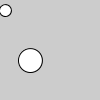Name

### mult()

Examples```v = PVector(5, 10, 0)
ellipse(v.x, v.y, 12, 12)
v.mult(6)
ellipse(v.x, v.y, 24, 24)
``````v1 = PVector(5, 10, 0)
ellipse(v1.x, v1.y, 12, 12)
v2 = PVector.mult(v1, 6)
ellipse(v2.x, v2.y, 24, 24)
```
Description Multiplies a vector by a scalar. The version of the method that uses a float acts directly on the vector upon which it is called (as in the first example above), and therefore has no return value. The versions that receive both a PVector and a float as arugments are static methods, and each returns a new PVector that is the result of the multiplication operation. Both examples above produce the same visual output.
Syntax
```.mult(n)
.mult(v, n)
.mult(v, n, target)
```
Parameters
n float: the number to multiply with the vector PVector: the vector to multiply by the scalar PVector: PVector in which to store the result

Updated on Mon Sep 21 15:53:25 2020.

If you see any errors or have comments, please let us know.